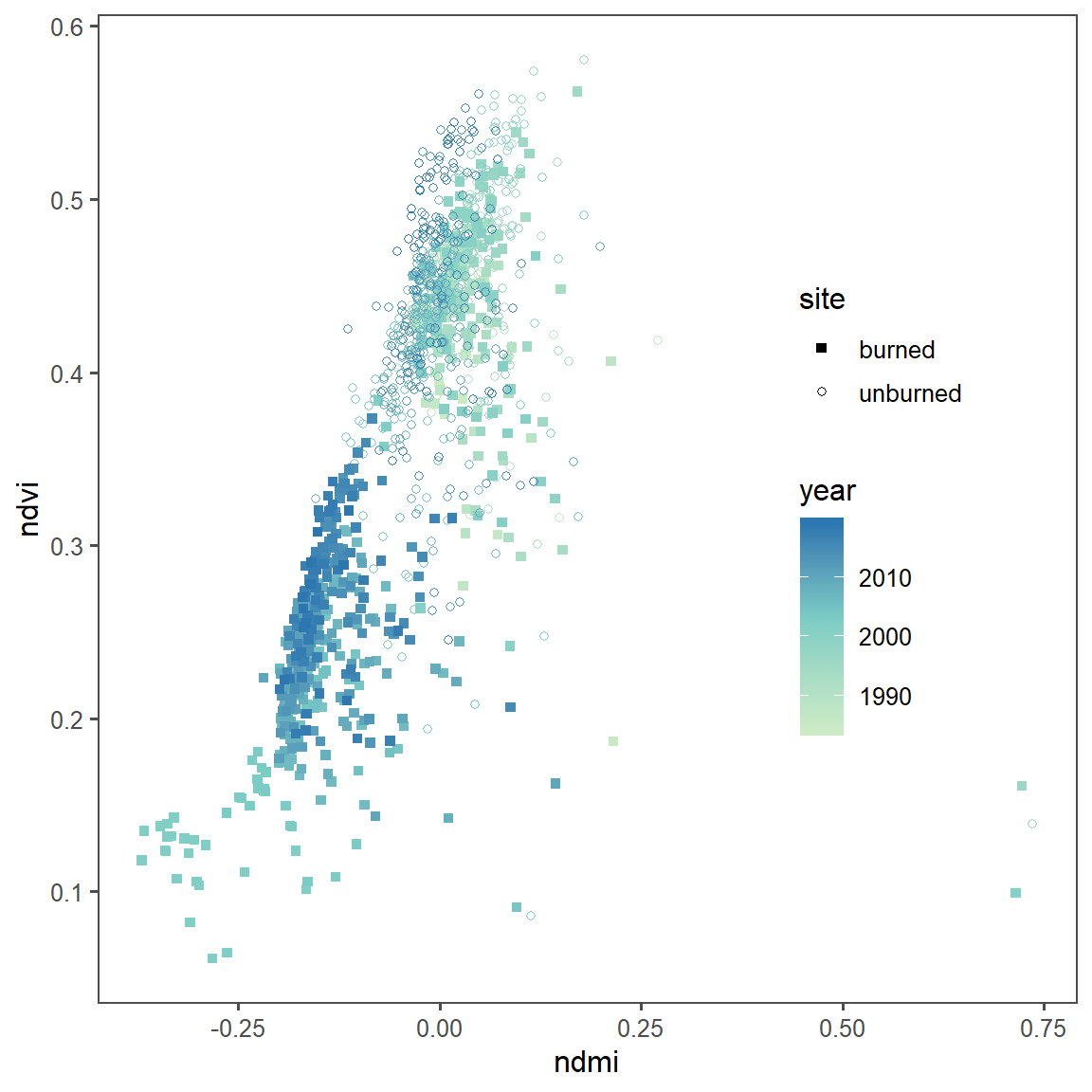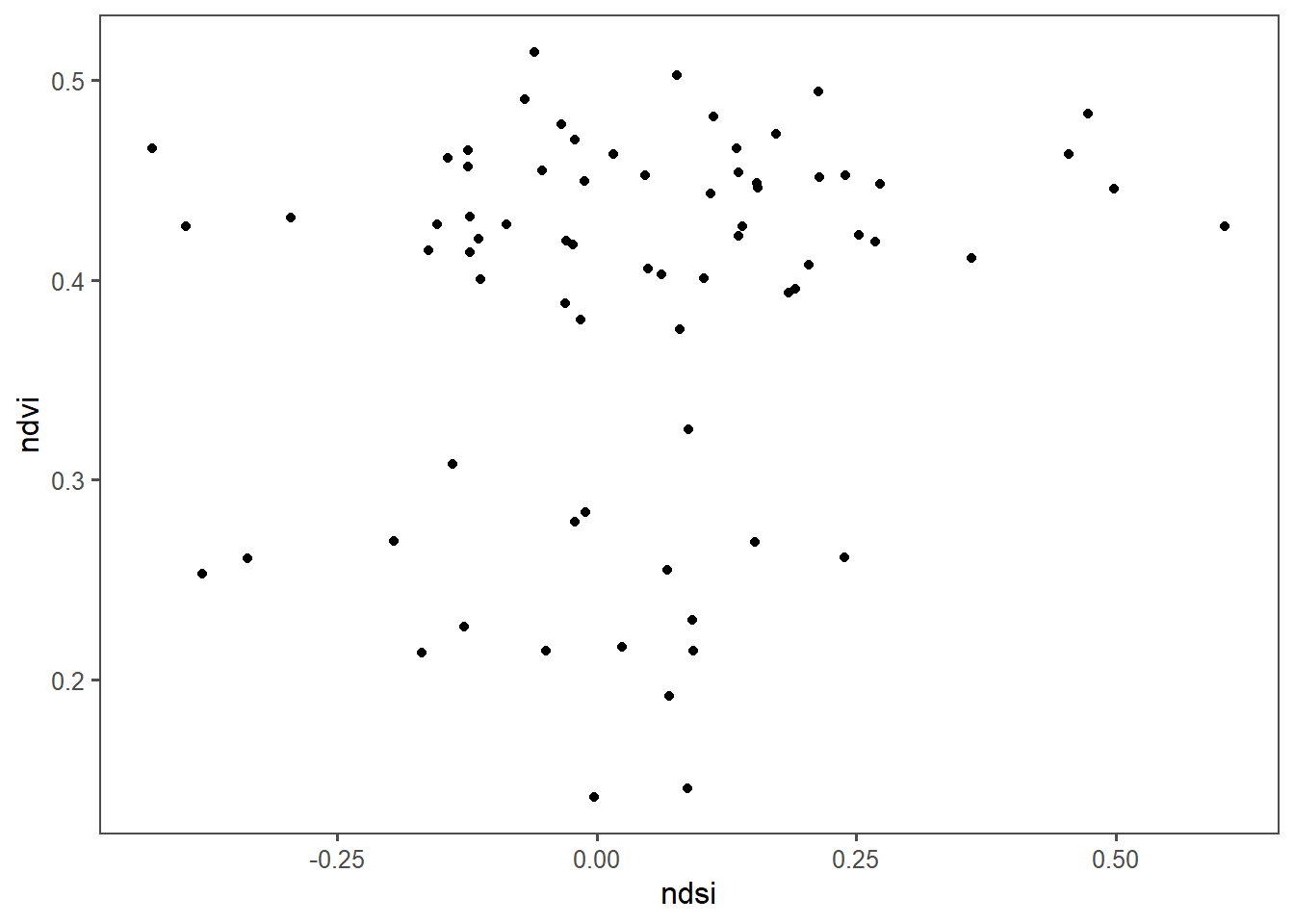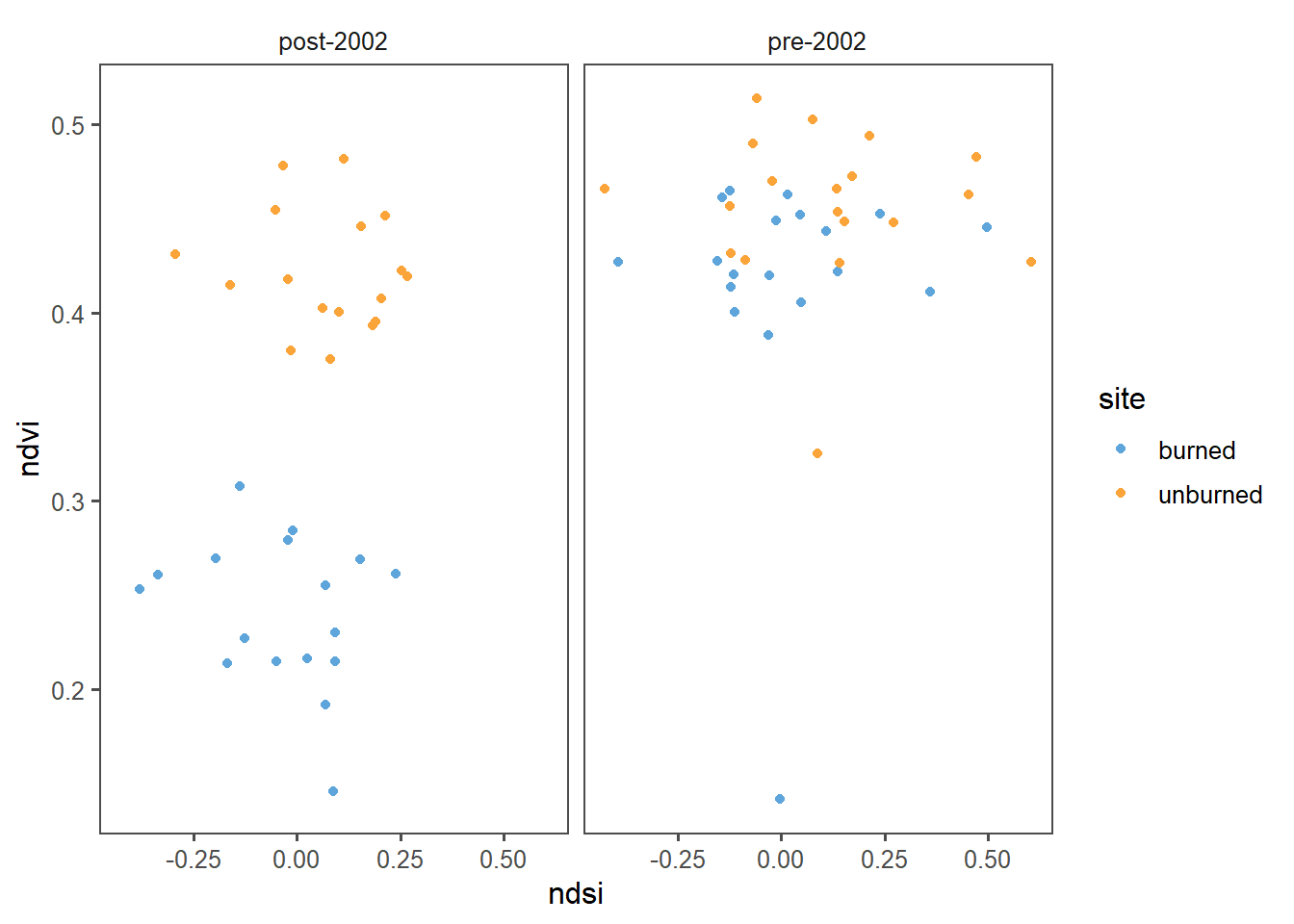library(tidyverse)
library(tidyr)
library(ggthemes)
library(lubridate)

# Now that we have learned how to munge (manipulate) data
# and plot it, we will work on using these skills in new ways

knitr::opts_knit\$set(root.dir='..')
####-----Reading in Data and Stacking it ----- ####
files <- list.files('data',full.names=T)

#Read in individual data files
ndmi <- read_csv(files) %>%
rename(burned=2,unburned=3) %>%
mutate(data='ndmi')

ndsi <- read_csv(files) %>%
rename(burned=2,unburned=3) %>%
mutate(data='ndsi')

rename(burned=2,unburned=3) %>%
mutate(data='ndvi')

# Stack as a tidy dataset
full_long <- rbind(ndvi,ndmi,ndsi) %>%
gather(key='site',value='value',-DateTime,-data) %>%
filter(!is.na(value))

## Question 1)

1 What is the correlation between NDVI and NDMI? - here I want you to convert the full_long dataset in to a wide dataset using the function “spread” and then make a plot that shows the correlation s a function of if the site was burned or not (x axis should be ndmi) You should exclude winter months and focus on summer months

### How I answered this question:

summer_only <- spread(full_long,key=data,value=value) %>%
filter(month(DateTime) %in% c(6,7,8,9)) %>%
mutate(year=year(DateTime))

ggplot(summer_only,aes(x=ndmi,y=ndvi,color=year,shape=site)) +
geom_point() +
scale_shape_manual(values=c(15,1)) +
theme_few() +
midpoint=2003) +
theme(legend.position = c(0.8,0.5))
## Warning: Removed 12 rows containing missing values (geom_point).### How some of you answered this question:

#### Making a summer only dataset with month and year columns

Notice how many of you actually did something that is more clear and better than what I did. By first making the wide dataset and then subsetting only to summer months, you have both a full dataset and a summer only one, which can save you operations down the road.

full_wide <- spread(full_long,key='data',value='value') %>%
filter_if(is.numeric,all_vars(!is.na(.))) %>%
mutate(month = month(DateTime), year = year(DateTime))

summer_only <- filter(full_wide, month %in% c(6,7,8,9))

ggplot(summer_only,aes(x=ndvi,y=ndmi,color=site)) +
geom_point() 

## Question 2

1. What is the correlation between average NDSI (normalized snow index) for January - April and average NDVI for June-August? In other words, does the previous year’s snow cover influence vegetation growth for the following summer?

### How I answered this question:

Notice here that I don’t join by site, because I wasn’t yet asking about site information (though I do in question 3!).

full_wide <- spread(data=full_long, key='data', value='value') %>%
filter_if(is.numeric, all_vars(!is.na(.))) %>%
mutate(month = month(DateTime),
year = year(DateTime))

winter_only <- filter(full_wide, month %in% c(1,2,3,4)) %>%
group_by(year,site) %>%
summarize(ndsi=mean(ndsi))

summer_only <- filter(full_wide, month %in% c(6,7,8)) %>%
group_by(year,site) %>%
summarize(ndvi=mean(ndvi))

winter_summer_together <- inner_join(winter_only, summer_only,
by = c('year','site'))

ggplot(winter_summer_together, aes(x=ndsi, y=ndvi)) +
geom_point() +
theme_few() +
scale_color_few() +
theme(legend.position=c(.2, .2))### How some of you answered this question:

Once again, y’all did it better than me. Here this person can directly use the same object from upstream (full_wide) and then subset from there. Minor note, with the select function you don’t have to (and shouldn’t) quote variable names (e.g. ‘site’ can be just site)

#Calculate average NDSI for winter months
ndsi_annual <- select(full_wide, 'site', 'ndsi', 'month', 'year') %>%
filter(month %in% c(1,2,3,4)) %>%
group_by(site,year) %>%
summarize(mean_NDSI=mean(ndsi))

#Calculate average NDVI for summer months
ndvi_annual <- select(full_wide, 'site', 'ndvi', 'month', 'year') %>%
filter(month %in% c(6,7,8)) %>%
group_by(site, year) %>%
summarise(mean_NDVI = mean(ndvi))

#Join NDSI and NDVI
annual_comparison <- inner_join(ndvi_annual, ndsi_annual, by = c('year', 'site'))

#Plot comparison between NDSI and NDVI
ggplot(annual_comparison, aes(x = mean_NDSI, y = mean_NDVI)) +
geom_point(colour = 'darkslategray4')+
xlab('Mean Annual Winter NDSI') +
ylab('Mean Annual Summer NDVI') +
ggtitle('Mean annual NDSI and NDVI comparison')+
theme_few()

## Q3

How is the snow effect from question 2 different between pre- and post-burn and burned and unburned?

### How I answered this question:

winter_summer_pre_post <- winter_summer_together %>%
mutate(status = ifelse(year > 2002,'post-2002','pre-2002'))

ggplot(winter_summer_pre_post,aes(x=ndsi,y=ndvi,color=site)) +
geom_point() +
theme_few() +
scale_color_few() +
facet_wrap(~status)### How some of you answered this question

Notice how you can use ifelse if a cut is only one cutting place (here 2003)

## This person had made an object called NDSI_NDVI (which is the same as
# winter_summer_pre_post)

NDSI_NDVI <- mutate(NDSI_NDVI, condition = cut(year, c(0, 2003, 2019),
labels = c("pre-burn", "post-burn")))

ggplot(NDSI_NDVI,aes(x=mean_ndsi,y=mean_ndvi,color=site)) +
geom_point() +
theme_few() +
facet_wrap(~condition) +
theme(legend.position=c(0.7,0.8)) 

## Question 4

What month is the greenest month on average?

### How I answered the question:

full_long %>%
filter(data == 'ndvi') %>% #Grab only the ndvi dataset
mutate(month=month(DateTime)) %>% #Add a month column
group_by(month) %>% #Group by that column
summarize(mean_ndvi = mean(value)) %>% #Take the mean of the ndvi (value) column
arrange(-mean_ndvi) #Arrange in descending order
## # A tibble: 12 x 2
##    month mean_ndvi
##    <dbl>     <dbl>
##  1     8     0.387
##  2     9     0.383
##  3     7     0.363
##  4    10     0.357
##  5     6     0.351
##  6     5     0.304
##  7    11     0.285
##  8    12     0.251
##  9     4     0.250
## 10     1     0.219
## 11     3     0.202
## 12     2     0.199

### How some of you answered the question

full_wide_monthly<- full_wide%>%
group_by(site, month)%>%
summarize(mean_ndvi=mean(ndvi),mean_ndsi=mean(ndsi))

ggplot(full_wide_monthly,aes(x=month,y=mean_ndvi,color=site)) +
geom_point()+
theme_few() +
scale_color_few() +
theme(legend.position=c(0.7,0.2))

greenest<-arrange(full_wide_monthly,desc(mean_ndvi))

## Question 5)

What month is the snowiest on average?

### How I answered the question:

full_long %>%
filter(data == 'ndsi') %>% #Grab only the ndvi dataset
mutate(month=month(DateTime)) %>% #Add a month column
group_by(month) %>% #Group by that column
summarize(mean_ndsi = mean(value)) %>% #Take the mean of the ndvi (value) column
arrange(-mean_ndsi) #Arrange in descending order
## # A tibble: 12 x 2
##    month mean_ndsi
##    <dbl>     <dbl>
##  1     1    0.210
##  2     2    0.199
##  3    12    0.124
##  4     3   -0.0420
##  5    11   -0.115
##  6     4   -0.228
##  7    10   -0.409
##  8     5   -0.411
##  9     6   -0.441
## 10     7   -0.450
## 11     9   -0.454
## 12     8   -0.459

### How some of you answered the question:

ggplot(full_wide_monthly,aes(x=month,y=mean_ndsi,color=site)) +
geom_point()+
theme_few() +
scale_color_few() +
theme(legend.position=c(0.7,0.7))

snowiest<-arrange(full_wide_monthly,desc(mean_ndsi))

snowiest